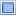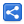# Java - Binary Search in Java

Binary Search, in general is a search algorithm which finds the index/position for a provided element value in an index based data structure like Java List and Array.

This algorithm look at the data structure at mid point to find the match, if match found at the mid point, the mid position is returned, otherwise left or right part of the middle is chosen based on target value is less than or greater than middle value. The mid point of the chosen part is again tested for a match. This process is continued until a match found.

The data structure must be sorted in natural order.

If elements are Java objects (not primitives) then they should either implement Comparable or should use a method which accepts a Comparator as parameter. If we use a Comparator it must order the elements in natural order, otherwise result will be unexpected.

## Examples

### Arrays.binarySearch() examples

There are number of overloaded binarySearch() static methods defined in Arrays class, for primitives and for object.`package com.logicbig.example;import java.util.Arrays;public class BinarySearchArrayExample {    public static void main (String[] args) {        int[] ints = {2, 1, 3, 4, 5};        Arrays.sort(ints);        System.out.printf("sorted array: %s%n", Arrays.toString(ints));        int index = Arrays.binarySearch(ints, 3);        System.out.println(index);    }}`

#### Output

`sorted array: [1, 2, 3, 4, 5]2``package com.logicbig.example;import java.util.Arrays;public class BinarySearchArrayExample2 {    public static void main (String[] args) {        String[] stringArray = {"one", "two", "three", "four"};        Arrays.sort(stringArray);        System.out.printf("sorted array: %s%n", Arrays.toString(stringArray));        int index = Arrays.binarySearch(stringArray, "three");        System.out.println(index);    }}`

#### Output

`sorted array: [four, one, three, two]2``package com.logicbig.example;import java.util.Arrays;import java.util.Comparator;public class BinarySearchArrayWithComparator {    public static void main (String[] args) {        MyObj[] array = {new MyObj(1), new MyObj(4), new MyObj(2), new MyObj(3)};        Comparator<MyObj> comp = (o1, o2) -> Integer.compare(o1.getI(), o2.getI());        Arrays.sort(array, comp);        int index = Arrays.binarySearch(array, new MyObj(2), comp);        System.out.println(index);    }    private static class MyObj {        private int i;        public MyObj (int i) {            this.i = i;        }        public int getI () {            return i;        }    }}`

`1`

## Collections.binarySearch() examples

Collections defines two static binarySearch() methods which only accept a List instance.`package com.logicbig.example;import java.util.Arrays;import java.util.Collections;import java.util.List;public class BinarySearchListExample {    public static void main (String[] args) {        List<String> list =                            Arrays.asList("one", "two", "three", "four");        Collections.sort(list);        System.out.println("sorted list: " + list);        int index = Collections.binarySearch(list, "three");        System.out.println(index);    }}`

#### Output

`sorted list: [four, one, three, two]2``package com.logicbig.example;import java.util.Arrays;import java.util.Collections;import java.util.Comparator;import java.util.List;public class BinarySearchListExample2 {    public static void main (String[] args) {        List<MyObj> list = Arrays.asList(new MyObj(2), new MyObj(1),                            new MyObj(4), new MyObj(3));        Comparator<MyObj> comp = (o1, o2) -> Integer.compare(o1.getI(), o2.getI());        list.sort(comp);        int index = Collections.binarySearch(list, new MyObj(4), comp);        System.out.println(index);    }    private static class MyObj {        private int i;        public MyObj (int i) {            this.i = i;        }        public int getI () {            return i;        }    }}`

#### Output

`3`

 Share